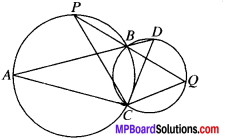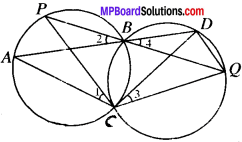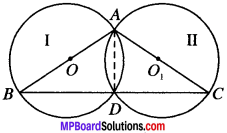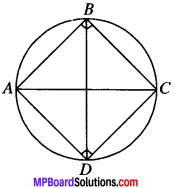# MP Board Class 9th Maths Solutions Chapter 10 Circles Ex 10.5

## MP Board Class 9th Maths Solutions Chapter 10 Circles Ex 10.5

Question 1.
In the figure, A, B and C are three points on a circle with centre O such that ∠BOC = 30° and ∠AOB = 60°. If D is a point on the circle other than the arc ABC, find ∠ADC.
Solution:
We have a circle with centre O, such that ∠AOB = 60° and ∠BOC = 30°∴ ∠AOB + ∠BOC = ∠AOC
∠AOB = 60° + 30° = 90°
Now, tne arc ABC subtends ∠AOC = 90° at the centre and ∠ADC at a point D on the circle other than the arc ABC.
∴ ∠ADC = $$\frac{1}{2}$$ [∠AOC]
∠ADC = $$\frac{1}{2}$$ (90°) = 45°Question 2.
A chord of a circle is equal to the radius of the circle. Find the angle subtended by the chord at a point on the minor arc and also at a point on the major arc.
Solution:
We have a circle having a chord AB equal to radius of the circle.
∴ AO = BO = AB
∆AOB is an equilateral triangle.
Since, each angle of an equilateral triangle = 60
∠AOB = 60°
Since, the arc ACB makes reflex ∠AOB = 360° – 60° = 300° at the centre of the circle and ∠ABC at a point on the minor arc of the circle.∠ACB = $$\frac{1}{2}$$ [reflex ∠AOB]
= $$\frac{1}{2}$$ [300°] = 150°
Similarly, ∠ADB = $$\frac{1}{2}$$ [∠AOB]
= $$\frac{1}{2}$$ x [60°] = 30°
Thus, the angle subtended by the chord on the minor arc = 150° and on the major arc = 30°.

Question 3.
In the figure, ∠PQR = 100°, where P, Q and R are points on a circle with centre O. Find ∠OPR.
Solution:
The angle subtended by an arc of a circle at its centre is twice the angle subtended by the same arc at a point on the circumference.∴ reflex ∠POR = 2∠PQR,
But ∠PQR = 100°
reflex ∠POR = 2 x 100° = 200°
Since, ∠POR + reflex ∠POR = 360°
∠POR + 200° = 360°
∠POR = 360° – 200°
∠POR = 160°
Since, OP = OR [Radii of the same circle]
∴ In ∆POR, ∠OPR = ∠ORP [Angles opposite to equal sides of a triangle are equal]
Also, ∠OPR + ∠ORP + ∠POR = 180° [Sum of the angles of a triangle = 180°]
∠OPR + ∠OPR + 160° = 180° [∴ ∠OPR = ∠ORP]
2∠OPR = 180° – 160° = 20°
∠OPR = $$\frac{20^{\circ}}{2}$$ =10°

Question 4.
In the Figure, ∠ABC = 69°, ∠ACB = 31°, find ∠BDC.
Solution:
We have, in ∆ABC,
∠ABC = 69° and
∠ACB = 31°But ∠ABC + ∠ACB + ∠BAC = 180°
∴ 69° +31° + ∠BAC = 180°
∠BAC = 180° – 69° – 31°
= 80°
Since, angles in the same segment are equal.
∴ ∠BDC = ∠BAC
∠BDC =80°

Question 5.
In the figure, A, B, C and D are four points on a circle. AC and BD intersect at a point E such that ∠BEC = 130° and ∠ECD = 20°. Find ∠BAC.
Solution:
In ∆CDE,
Exterior ∠BEC – ∠AED = 130°130° = ∠EDC + ∠ECD
130° = ∠EDC + 20°
∠EDC = 130° – 20° = 110°
∠BDC = 110°
Since, angles in the same segment are equal.
∠BAC = ∠BDC
∠BAC = 110°Question 6.
ABCD is a cyclic quadrilateral whose diagonals intersect at a point E. ∠DBC = 70°, ∠BAC is 30°, find ∠BCD. Further, if AB = BC, find ∠ECD.
Solution:
Given:
∠BAC = 30°, ∠DBC = 70°
To find:
∠BCD and ∠ECD.
∠BDC = ∠BAC = 30° (∠s on the same segment of a circle are equal)In ∆BCD, 70° + 30° + ∠C = 180° (ASP)
100° + ∠C = 180°
∠C = 80°
AB = BC (Given)
∠BAC = ∠BCA = 30°
∠BCD = ∠BCA + ∠ECD
80° = 30° + ∠ECD
∠ECD – 50°

Question 7.
If diagonals of a cyclic quadrilateral are diameters of the circle through the vertices of the quadrilateral, prove that it is a rectangle.
Solution:
Given
ABCD is a cyclic quadrilateral in which AC and BD are diagonals.
To prove:
ABCD is a rectangle.Proof:
BD is the diameter of the circle.
∠BAD = 90°(angle in a semicircle)
Similarly ∠BCD = 90°
AC is the diameter of the circle
∠ABC = 90° (angle in a semicircle)
In quadrilateral ABCD, ∠A = ∠B = ∠C = ∠D = 90°
ABCD is a rectangle.

Question 8.
If the non-parallel sides of h trapezium are equal, prove that it is cyclic.
Solution:
Given:
AD = BC, AB ∥ DC.
To prove:
Construction:
Draw AE and BF As on DC.Proof:
∴ AE = BF
(∴ Distance between two parallel lines are equal)
∠E = ∠F (Each 90°)
and so ∠D = ∠C (By CPCT)
AB ∥ DC and AD is the transversal.Question 9.
Two circles intersect at two points B and C. Through B, two line segments ABD and PBQ are J drawn to intersect the circles at A, D and Q respectively (see Fig.). Prove that ∠ACP = ∠QCD.Solution:
Given
C (O, r) and C (O1, r1) are two circles. Two lines ABD and PBQ are drawn which intersect at B.
To prove:
∠1 = ∠3Proof:
∠1 = ∠2 …(1) (Z s on the same segment of a circle are equal.)
∠3 = ∠4 …..(2) (Z s on the same segment of a circle are equal)
∠2 = ∠4 …(3) (OA’s)
From (1), (2) and (3), we get
∠1 = ∠3 …(2)

Question 10.
If circles are drawn taking two sides of a triangle as diameters, prove that the point of intersection of these circles lie on the third side.
Solution:
Given
C (O, r) and C (O1, r1) are two Circles in whichAB andAC are diameter. These circles intersect at point A and D.
To prove:
BDC is a line.
Proof:
∠ADB = 90° (∠s in a semicircle)
∠ADC) = 90° (∠s in a semicircle)Adding (1) and (2), we get
∠BDC = 180°
∴ BDC is a line and hence D lies on the third side.

Question 11.
ABC and ADC are two right triangles with common hypotenuse AC. Prove that ∠CAD – ∠CBD.
Solution:
Given:
ABC and ADC are two right ∆’s on common base AC. ∠B – 90° and ∠D = 90°.
Proof:
∠ABC + ∠ADC = 90° + 90°
= 180°∠CAD and ∠CBD are angles on the same segment of a circle.Question 12.
Prove that a cyclic parallelogram is a rectangle.
Solution:
Given: ABCD is a ∥gm
To prove: ABCD is a rectangle.Proof:
∠A = ∠C
∠A + ∠C =180°
∠A + ∠A = 180°
∠A = $$\frac{180^{\circ}}{2}$$ = 90°
∴ ABCD is a rectangle.
∠A = $$\frac{180^{\circ}}{2}$$ = 90°
∴ ABCD is a rectangle.